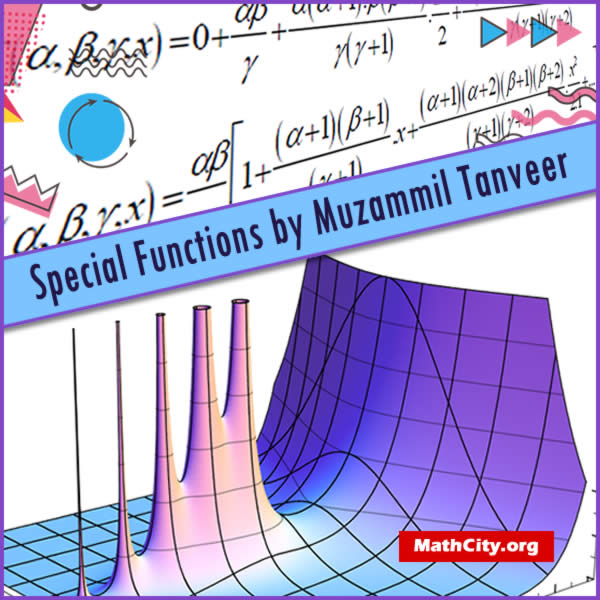Special Functions by Dr. Muhey-U-Din

These notes are provided and composed by Mr. Muzammil Tanveer. We are really very thankful to him for providing these notes and appreciates his effort to publish these notes on MathCity.org. Thease notes are based on the lectures by Dr. Muhey-U-Din.Name Special Functions Mr. Muzammil Tanveer Dr. Muhey-U-Din 119 pages PDF (see Software section for PDF Reader) 853 kB
• Gamma Function
• Some Properties of Gamma Function
• Beta Function
• Duplication Formula
• Hypergeometric Function
• Historical Background of Hypergeometric Function
• Hypergeometric function of one variable
• Hypergeometric equation
• Symmetric property of Hypergeometric Function
• Differentiation of Hypergeometric Function
• Integral representation of Hypergeometric Function
• Gauss Theorem
• Pochhammer symbol
• Confluent Hypergeometric Differential Equation
• Solution of Confluent Hypergeometric function
• Recurrence relation of Confluent Hypergeometric function
• Differentiation of Confluent Hypergeometric function
• Integral representation of Confluent Hypergeometric function
• Kummer’s Relation
• Application of Confluent Hypergeometric function
• Hermite’s Equation
• Solution of Hermite’s Equation
• Recurrence relation of Hermite equation
• Generation function of Hermite polynomials
• Orthogonality property
• Laguerre’s Function
• Generating function for Laguerre’s polynomial
• Bessel’s Function
• Solution of Bessel’s Differential Equation
• Recurrence formula of Bessel Function
• Rodrigues Formula
• Special results involving Legendre polynomial
• Legendre’s Equation
• Solution of Legendre’s Equation
• Some Modern Special Functions
• Wright Function
• Normalized form of Wright Function
• Mittag-Leffler Function
• Normalized form of Mittag-Leffler Function

Please click on View Online to see inside the PDF.

• msc/notes/special-functions-muzammil-tanveer